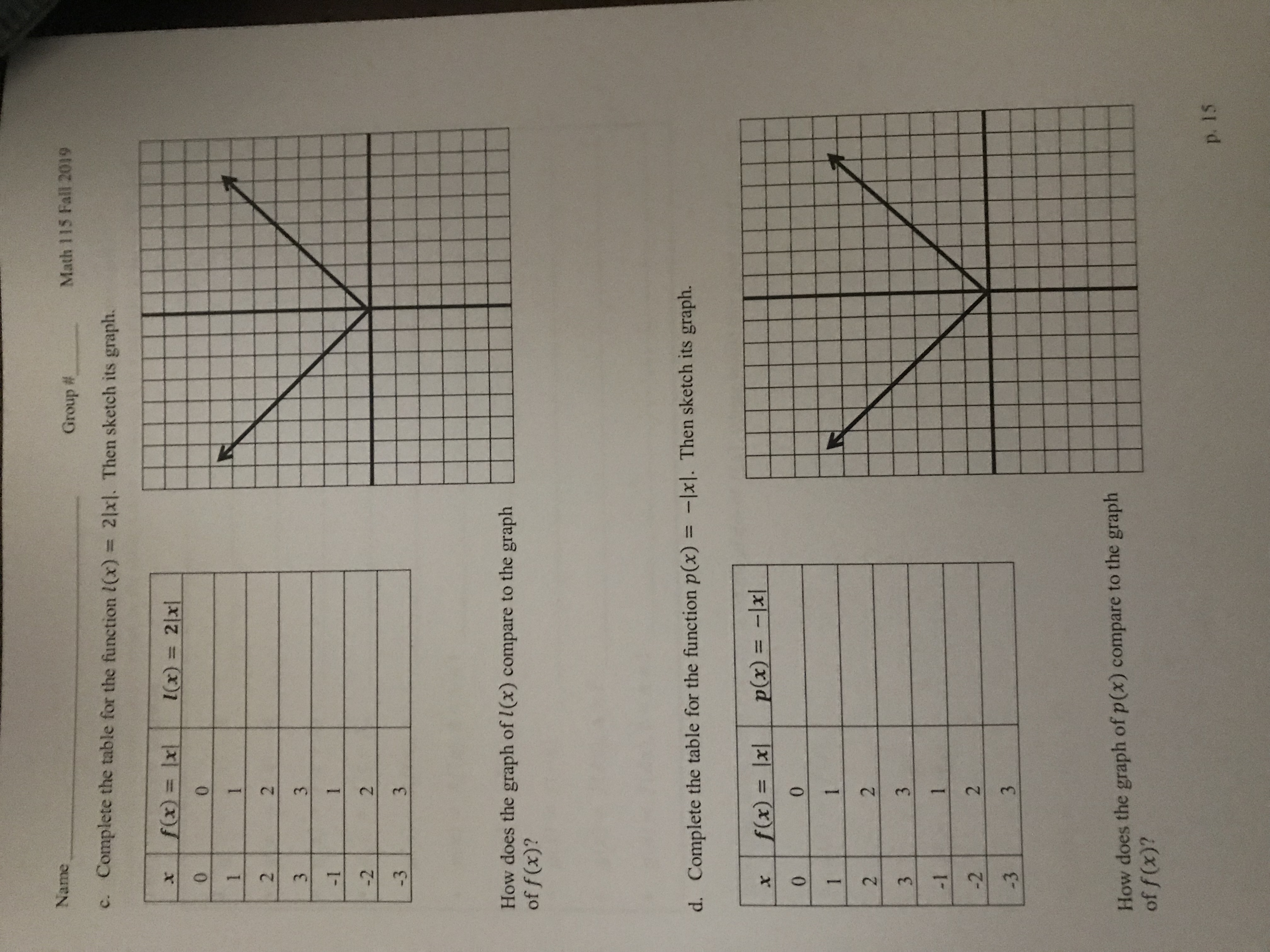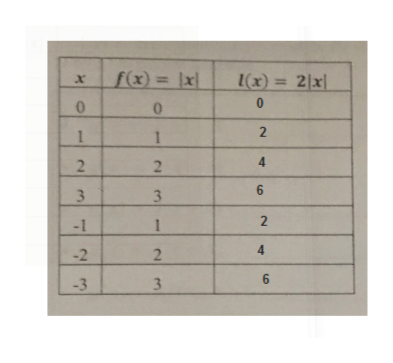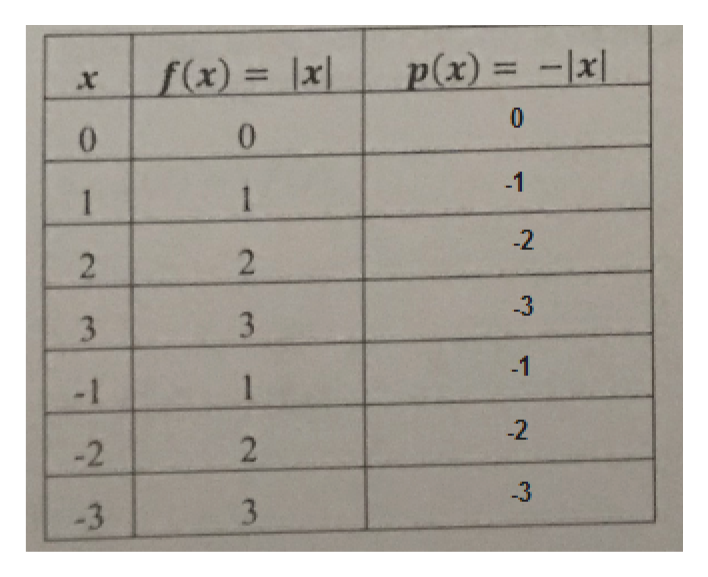# NameMath 115 Fall 2019Group #Complete the table for the function l(x) = 21x]. Then sketch its graph.C.f(x) = lxlx) 2lxX00112231-12-23-3How does the graph of /(x) compare to the graphof f(x)?d. Complete the table for the function p(x) = -xl. Then sketch its graph.p(x)=-lxf(x) = |x|X00112231-12-23-3How does the graph of p(x) compare to the graphof f (x)?p. 15r)

Question

problem 1- transformation of the absolute value fuctions: For each part below, the table of the values and the graph for f(x)= |x| is given. complete the table and sketch the grapg for the given function, and compare it to function f.help_outlineImage TranscriptioncloseName Math 115 Fall 2019 Group # Complete the table for the function l(x) = 21x]. Then sketch its graph. C. f(x) = lx lx) 2lx X 0 0 1 1 2 2 3 1 -1 2 -2 3 -3 How does the graph of /(x) compare to the graph of f(x)? d. Complete the table for the function p(x) = -xl. Then sketch its graph. p(x)=-lx f(x) = |x| X 0 0 1 1 2 2 3 1 -1 2 -2 3 -3 How does the graph of p(x) compare to the graph of f (x)? p. 15 r) fullscreen
check_circle

Step 1

We have plugged values of x in the function l(x)=2|x| and completed the table.help_outlineImage Transcriptionclosef(x) = \xl (x) 2x X 10 2 1 4 2 2 3 2 -1 -2 2 3 -3 fullscreen
Step 2

Then plotted the points and joined them with lines.

f(x) has been vertically stretched by 2 to form l(x). And values of f(x) was multiplied by 2 to get values of l(x)

Step 3

We have plugged values of x in the function p...help_outlineImage Transcriptionclosep(x) = -lx f(x) = |x| 1 x 0 0 0 -1 1 -2 2 -3 3 3 -1 1 - 1 -2 -2 -3 3 2 2/ 3 1 fullscreen

### Want to see the full answer?

See Solution

#### Want to see this answer and more?

Solutions are written by subject experts who are available 24/7. Questions are typically answered within 1 hour.*

See Solution
*Response times may vary by subject and question.
Tagged in

### Functions